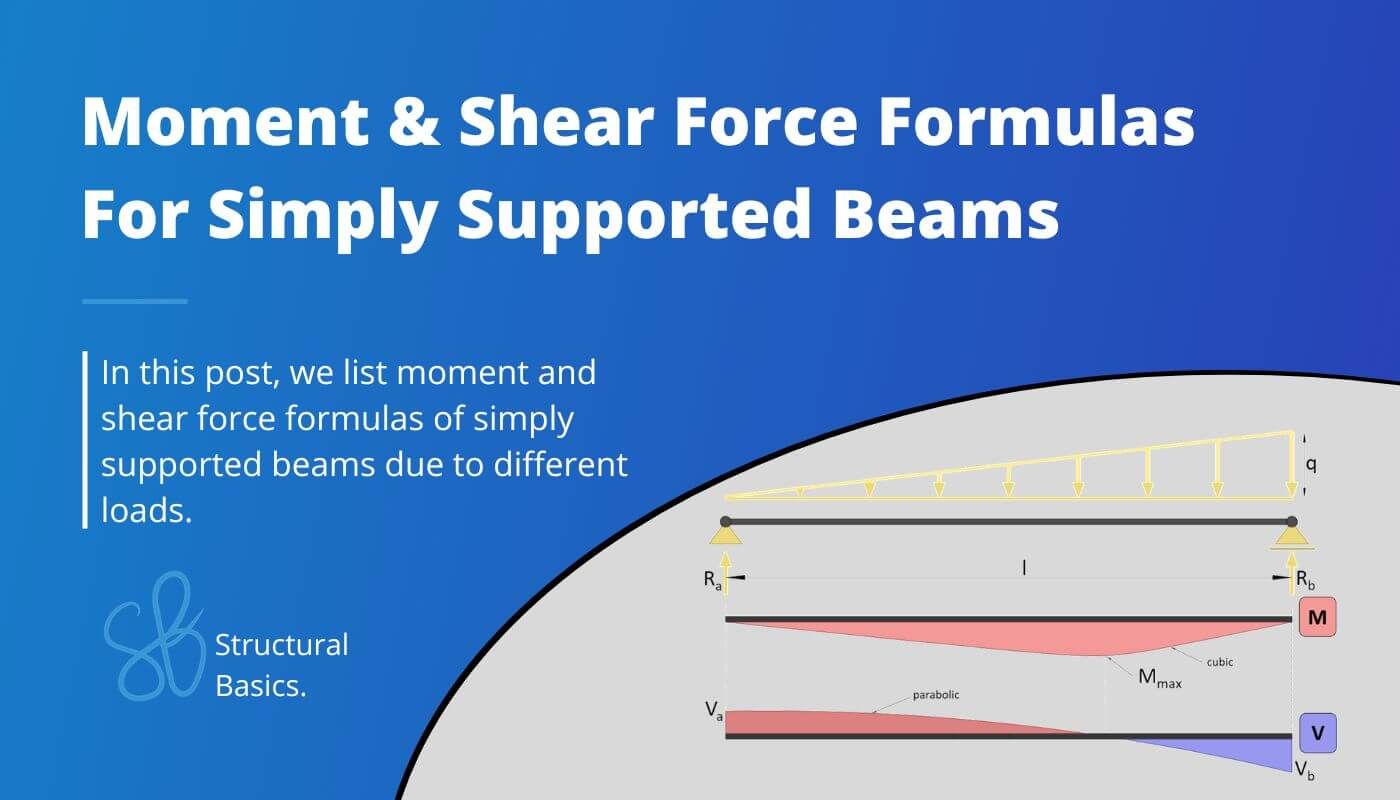# Simply Supported Beam – Moment & Shear Force Formulas Due To Different LoadsLast updated: June 6th, 2023

Calculating bending moments and shear forces in beams for different loading scenarios is probably one of the things in structural engineering that we do throughout our studies and also careers later on.

While it’s very important to know how to derive and calculate the internal forces, the further we get in our studies, the more we can use beam moment and shear formulas.

In this post we’ll show, the most important and easiest formulas for simply supported beams due to different loading scenarios like UDL line loads, point loads and Triangular loads.

All will be shown and explained by examples.

Now, before we get started, always remember that the unit of the bending moment is Kilonewton meter [$kNm$] and Kilonewton [$kN$] for the shear forces when in Europe.

But now, let’s get started.

## 1. Simply supported beam – uniformly distributed line load (UDL) formulas

### Bending moment

$M(x) = 1/2 \cdot q \cdot x \cdot (l-x)$

### Max bending moment

$M_{max} = 1/8 \cdot q \cdot l^2$

### Shear forces at supports

$V_{a} = -V_b = 1/2 \cdot q \cdot l$

### Reaction forces

$R_a = R_b = 1/2 \cdot q \cdot l$

Those formulas can also be calculated by hand. Check out this article if you want to learn in depth how to calculate the bending moments, shear and reaction forces by hand.

## 2. Simply supported beam – Uniformly distributed load (UDL) at midspan (formulas)

### Max bending moment

$M_{max} = \frac{q\cdot b}{l^2} \cdot (c+\frac{b}{2})\cdot (a+\frac{b}{2})\cdot (l- \frac{b}{2})$

if $x = a + \frac{b\cdot (c + \frac{b}{2})}{l}$

### Shear forces at supports

$V_{a} = q \cdot b \cdot \frac{c + \frac{b}{2}}{l}$

$V_{b} = -q \cdot b \cdot \frac{a + \frac{b}{2}}{l}$

### Reaction forces

$R_{a} = q \cdot b \cdot \frac{c + \frac{b}{2}}{l}$

$R_{b} = q \cdot b \cdot \frac{a + \frac{b}{2}}{l}$

## 3. Simply supported beam – Uniformly distributed load (UDL) at 1 support (formulas)

### Max bending moment

$M_{max} = 1/2 \cdot q \cdot a^2 \cdot (1 – \frac{a}{2l})^2$

if $x = a \cdot (1- \frac{a}{2l})$

### Shear forces at supports

$V_{a} = q \cdot a \cdot (1-\frac{a}{2l})$

$V_{b} = -q \cdot a \cdot \frac{a}{2l}$

### Reaction forces

$R_{a} = q \cdot a \cdot (1-\frac{a}{2l})$

$R_{b} = q \cdot a \cdot \frac{a}{2l}$

## 4. Simply supported beam – Point load at midspan (formulas)

### Bending moment

$M(x) = 1/2 \cdot Q \cdot x$ if x < l/2

$M(x) = 1/2 \cdot Q \cdot (l- x)$ if x > l/2

### Max bending moment

$M_{max} = 1/4 \cdot Q \cdot l$

### Shear forces at supports

$V_{a} = 1/2 \cdot Q$

$V_{b} = -1/2 \cdot Q$

### Reaction forces

$R_{a} = 1/2 \cdot Q$

$R_{b} = 1/2 \cdot Q$

## 5. Simply supported beam – Point load not at midspan (formulas)

### Bending moment

$M(x) = Q \cdot b \cdot \frac{x}{l}$ if x < a

$M(x) = Q \cdot a \cdot \frac{l-x}{l}$ if x > a

### Max bending moment

$M_{max} = Q \cdot \frac{a\cdot b}{l}$

### Shear forces at supports

$V_{a} = Q \cdot \frac{b}{l}$

$V_{b} = -Q \cdot \frac{a}{l}$

### Reaction forces

$R_{a} = Q \cdot \frac{b}{l}$

$R_{b} = Q \cdot \frac{a}{l}$

## 6. Simply supported beam – 2 Point loads – equally spaced (formulas)

### Bending moment

$M(x) = Q \cdot x$ if x < a

### Max bending moment

$M_{max} = Q \cdot a$

### Shear forces at supports

$V_{a} = Q$

$V_{b} = -Q$

### Reaction forces

$R_{a} = Q$

$R_{b} = Q$

## 7. Simply supported beam – 3 Point loads – equally spaced (formulas)

### Bending moment

$M(x) = 3/2 Q \cdot x$ if x < l/4

### Max bending moment

$M_{max} = 1/2 \cdot Q \cdot l$

### Shear forces at supports

$V_{a} = 3/2 \cdot Q$

$V_{b} = -3/2 \cdot Q$

### Reaction forces

$R_{a} = 3/2 \cdot Q$

$R_{b} = 3/2 \cdot Q$

## 8. Simply supported beam – 2 Point loads – unequally spaced (formulas)

### Bending moment M1

$M = \frac{Q}{l} \cdot (l – a + b) \cdot a$ max Moment if a>b

### Max bending moment M2 (max Moment if a<b)

$M_{max} = \frac{Q}{l} \cdot (l – b + a) \cdot b$

### Shear forces at supports

$V_{a} = \frac{Q}{l} \cdot (l – a + b)$

$V_{b} = -\frac{Q}{l} \cdot (l – b + a)$

### Reaction forces

$R_{a} = \frac{Q}{l} \cdot (l – a + b)$

$R_{b} = \frac{Q}{l} \cdot (l – b + a)$

## 9. Simply supported beam – One side triangular line load (formulas)

### Bending moment

$M(x) = \frac{1}{6} \cdot q \cdot l \cdot x \cdot (1- (\frac{x}{l})^2)$

### Max bending moment

$M_{max} = 0.064 \cdot q \cdot l^2$ at $x = \frac{1}{\sqrt{3}}\cdot l$

### Shear forces at supports

$V_{a} = \frac{1}{6} \cdot q \cdot l$

$V_{b} = -\frac{1}{3} \cdot q \cdot l$

### Reaction forces

$R_{a} = \frac{1}{6} \cdot q \cdot l$

$R_{b} = \frac{1}{3} \cdot q \cdot l$

## 10. Simply supported beam – Double Triangular line load (formulas)

### Bending moment

$M(x) = \frac{1}{4} \cdot q \cdot l \cdot x \cdot (1- \frac{4}{3} \cdot (\frac{x}{l})^2)$ for 0 < x < l/2

### Max bending moment

$M_{max} = \frac{1}{12} \cdot q \cdot l^2$

### Shear forces at supports

$V_{a} = \frac{1}{4} \cdot q \cdot l$

$V_{b} = -\frac{1}{4} \cdot q \cdot l$

### Reaction forces

$R_{a} = \frac{1}{4} \cdot q \cdot l$

$R_{b} = \frac{1}{4} \cdot q \cdot l$

If you are new to structural design, then check out our design tutorials where you can learn how to use the calculated bending moments and shear forces to design structural elements such as

Do you miss any Moment or shear force formulas for the simply supported beam that we forgot in this article? Let us know in the comments✍️